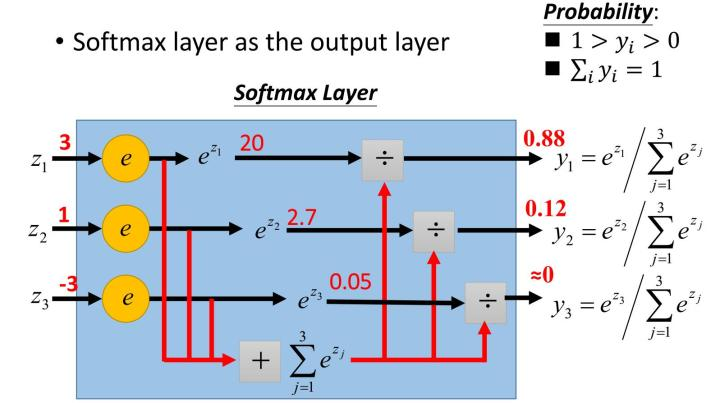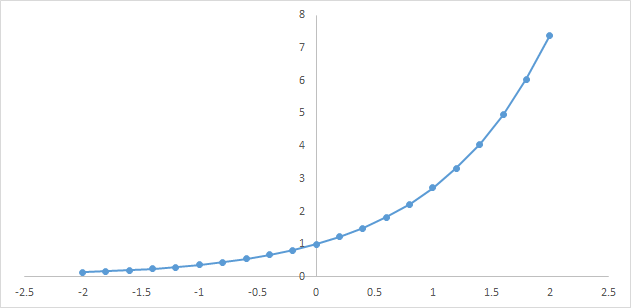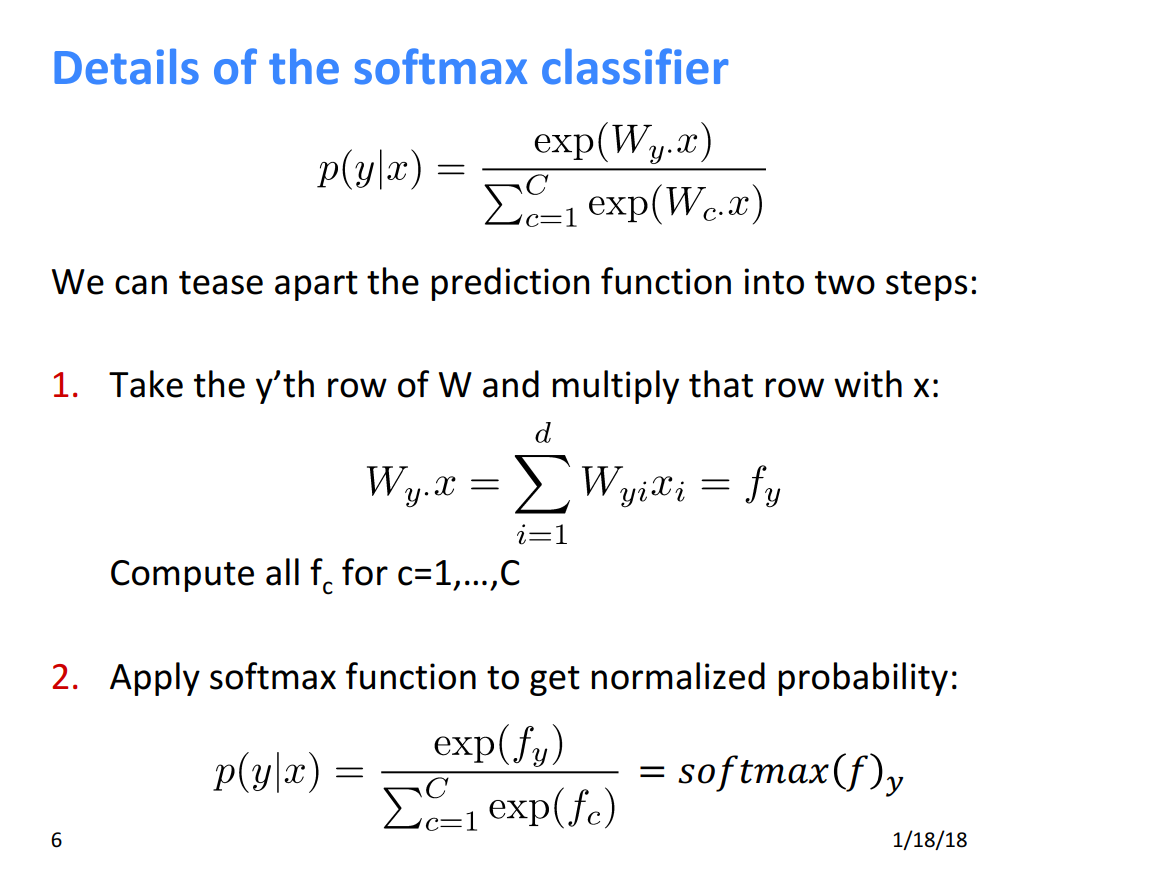# 一分钟理解softmax函数（超简单）softmax就是将在负无穷到正无穷上的预测结果按照这两步转换为概率的。

1）将预测结果转化为非负数2）各种预测结果概率之和等于1

1）将预测结果转化为非负数

y1 = exp(x1) = exp(-3) = 0.05

y2 = exp(x2) = exp(1.5) = 4.48

y3 = exp(x3) = exp(2.7) = 14.88

2）各种预测结果概率之和等于1

z1 = y1/(y1+y2+y3) = 0.05/(0.05+4.48+14.88) = 0.0026

z2 = y2/(y1+y2+y3) = 4.48/(0.05+4.48+14.88) = 0.2308

z3 = y3/(y1+y2+y3) = 14.88/(0.05+4.48+14.88) = 0.7666

1）分子：通过指数函数，将实数输出映射到零到正无穷。

2）分母：将所有结果相加，进行归一化。09-163571

#### Softmax 函数的特点和作用（转载）03-037061

#### Python实现softmax函数

09-193万+

#### 详解sigmoid与softmax， 多分类及多标签分类

08-019885

#### 深度学习之softmax函数（一）

09-0229万+

#### 小白都能看懂的softmax详解

03-214468

#### 详解softmax函数以及相关求导过

07-141373

#### softmax函数的正推原理——softmax前世今生系列(1)

08-112535

#### CNN入门讲解：我的Softmax和你的不太一样

11-277525

#### 斯坦福大学深度学习公开课cs231n学习笔记（1）softmax函数理解与应用©️2020 CSDN 皮肤主题: 精致技术 设计师: CSDN官方博客点击重新获取扫码支付1.余额是钱包充值的虚拟货币，按照1:1的比例进行支付金额的抵扣。
2.余额无法直接购买下载，可以购买VIP、C币套餐、付费专栏及课程。余额充值